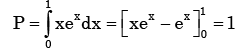Courses

# Calculus, Theorems Of Integral Calculus - MCQ Test 2

## 20 Questions MCQ Test Mock Test Series for Civil Engineering (CE) GATE 2020 | Calculus, Theorems Of Integral Calculus - MCQ Test 2

Description
This mock test of Calculus, Theorems Of Integral Calculus - MCQ Test 2 for Mechanical Engineering helps you for every Mechanical Engineering entrance exam. This contains 20 Multiple Choice Questions for Mechanical Engineering Calculus, Theorems Of Integral Calculus - MCQ Test 2 (mcq) to study with solutions a complete question bank. The solved questions answers in this Calculus, Theorems Of Integral Calculus - MCQ Test 2 quiz give you a good mix of easy questions and tough questions. Mechanical Engineering students definitely take this Calculus, Theorems Of Integral Calculus - MCQ Test 2 exercise for a better result in the exam. You can find other Calculus, Theorems Of Integral Calculus - MCQ Test 2 extra questions, long questions & short questions for Mechanical Engineering on EduRev as well by searching above.
QUESTION: 1

### A function is given by f(t) = sin2t + cos 2t. Which of the following is true?

Solution: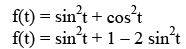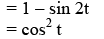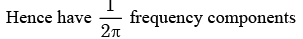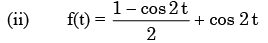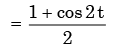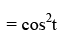QUESTION: 2

### Following are the values of a function y(x) : y(-1) = 5, y(0), y(1)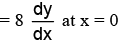as per Newton’s central  difference scheme is:

Solution: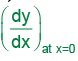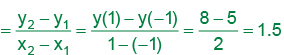QUESTION: 3

###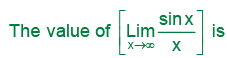Solution: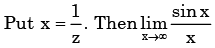QUESTION: 4

The function f(x) = |x+1| on the interval [-2, 0]

Solution:

f(x ) = x+ 1

f is continuous in [−2, 0]

but not differentiable at

x =−1 because we can draw

infinite number of tangents at x = −1

QUESTION: 5

If y=|x| for x<0 and y=x for x ≥ 0, then

Solution: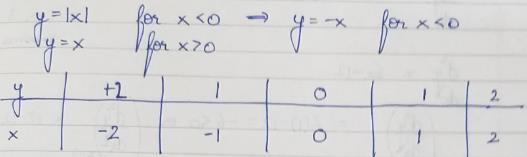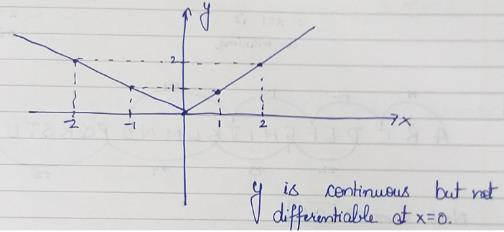QUESTION: 6

What is the derivative of f(x) = |x| at x = 0?

Solution:

f( x ) = x .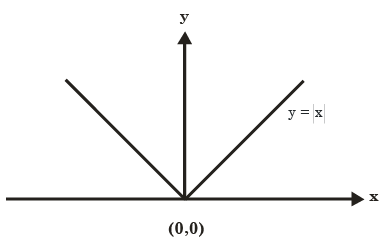At x = 0, we can draw infinitely many tangents at x=0.So limit does not exists.

QUESTION: 7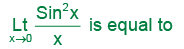Solution: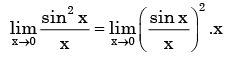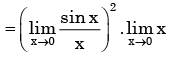= 1.0= 1

QUESTION: 8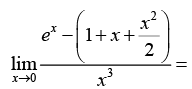Solution: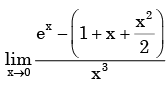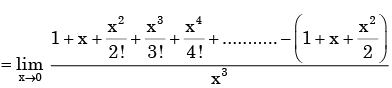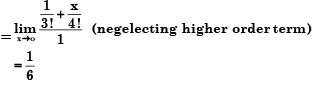QUESTION: 9

The function Y=| 2-3x |

Solution:
QUESTION: 10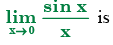Solution:
QUESTION: 11

The function f(x) = x3 - 6x2 + 9x + 25 has

Solution: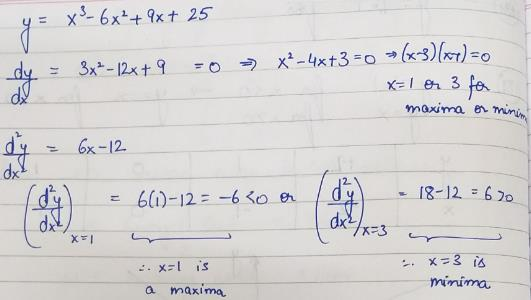QUESTION: 12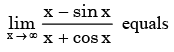Solution: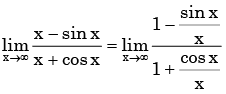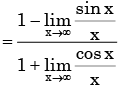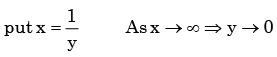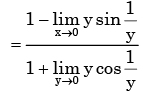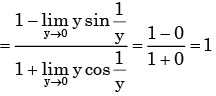QUESTION: 13

The value of the integral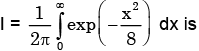Solution: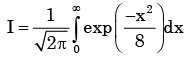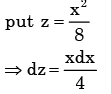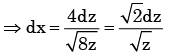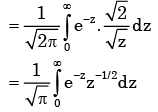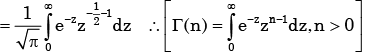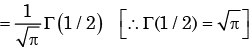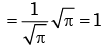QUESTION: 14

The following plot shows a function y which varies linearly with x. The value of the integral I =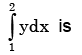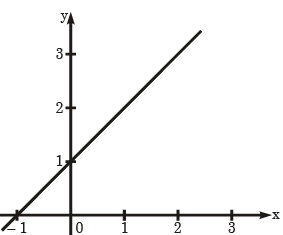Solution:

Here the points (0,1) and (-1,0) are on the time

∴The equn of the line is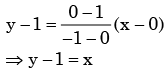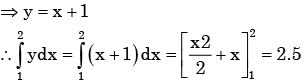QUESTION: 15

The value of the integral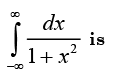Solution: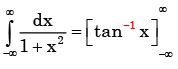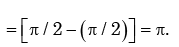QUESTION: 16

The length of the curve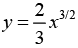between x = 0 and x = 1 is

Solution:

Length of  the wire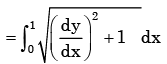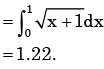QUESTION: 17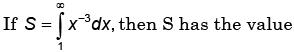Solution: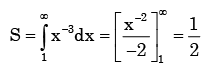QUESTION: 18

A continuous-time system is described by y (t) = e − x (t) where y (t) is the output and x (t) is the input. y(t) is bounded.

Solution: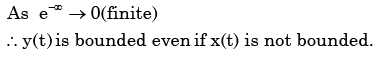QUESTION: 19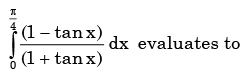Solution:

Since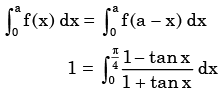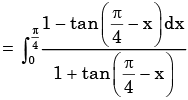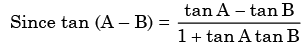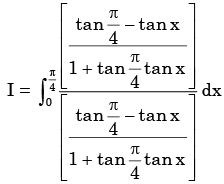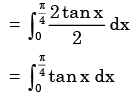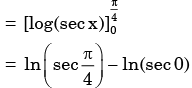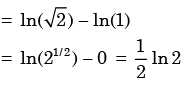QUESTION: 20

The value of the quantity P, where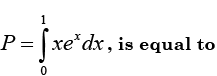Solution: